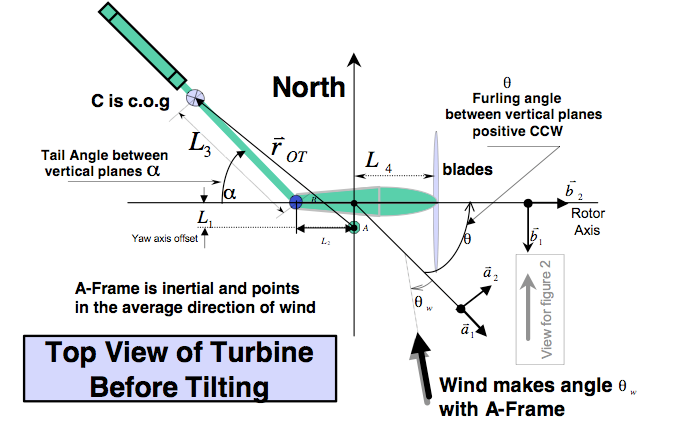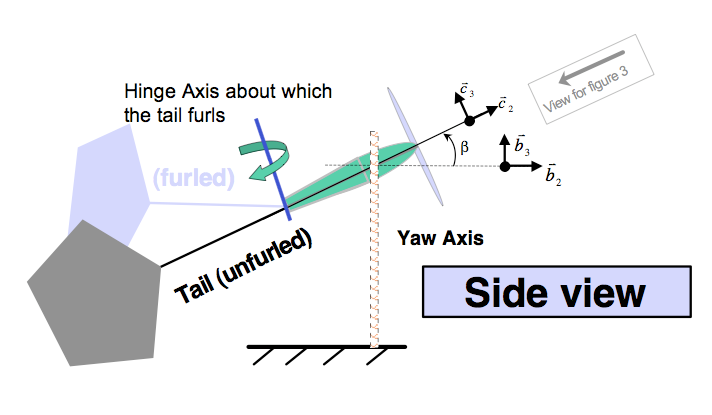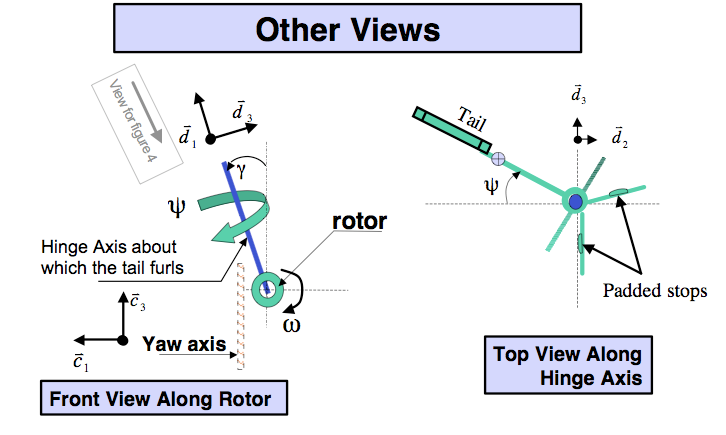User Name Remember Me? Password

 Kinematics and Dynamics Kinematics and Dynamics Physics Help ForumDec 5th 2008, 05:41 PM #1 Junior Member   Join Date: Dec 2008 Posts: 1 reference frame difficulty 1. The problem statement, all variables and given/known data This is a basic question to a kind of complex problem, any help will be deeply appreciatted. I have all the motion equations for the system described below, but i have a problem with the reference frames... So the problem is as follows: A small wind generator is protected against high speed winds by autofurling mechanism that depends on the equilibrium of torques between the tail and the moment of inertia generated by wind when it hits the rotor: the diagramsTHE COORDINATE FRAMES 1. inertial frame F_A=a_1, a_2, a_3 point in the average direction of the wind. 2 F_bframe of reference attached to nacelle before tilting b_1 = a_1 cos (theta) + a_2 sin (theta) b_2 = - a_1 sin (theta) + a_2 cos(theta) b_3 = a_3 vertical upward 3 F_C=c_1, c_2, c_3 after tilting 4 F_D = d_1, d_2 ,d_3 aligned with the tail hinge 2. Relevant equations What are the equations for the different the different coordinate systems?? i have the equations for the coordinate system Fb, but when i derived them following the diagrams i got different relations, are the equations given to me wrong or what i'm doing is wrong? 3. The attempt at a solution So for the coordinate system F_B, according to the diagrams i get that: b_1 =a_1 sin (theta) - a_2 cos(theta) b_2 =a_1 cos(theta) + a_2 sin(theta) b_3 = a_3 since i don't get the same result for reference frame b, i'm prety doubtful of what i get in c and d. Thanks in advance... Last edited by chicomore; Dec 5th 2008 at 05:47 PM.Tags difficulty, frame, referenceSearch tags for this page

### system of reference physics forum

Click on a term to search for related topics.
 Thread ToolsShow Printable VersionEmail this Page Display ModesLinear ModeSwitch to Hybrid ModeSwitch to Threaded ModeSimilar Physics Forum Discussions Thread Thread Starter Forum Replies Last Post PhysicsKidd Kinematics and Dynamics 3 Nov 5th 2014 01:13 PM avengefulghost Kinematics and Dynamics 1 Jan 16th 2011 07:56 PM mohabitar Kinematics and Dynamics 1 Sep 8th 2010 10:16 AM HassanZahid Special and General Relativity 1 Dec 24th 2008 04:35 PM evabern Special and General Relativity 1 Oct 15th 2008 10:58 AM